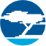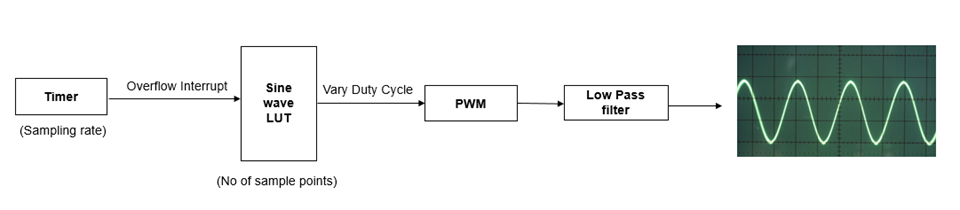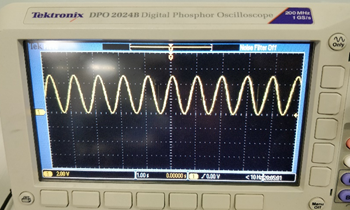cancel
Showing results for
Did you mean:

Generating Sine Wave using PWM in PSoC – KBA226852Employee

Generating Sine Wave using PWM in PSoC – KBA226852

Translation - Japanese:

Question:

How do you generate a sine wave using PWM in PSoC®?

Sine wave is generated by varying the duty cycle of the pulse width modulation (PWM) signal at regular intervals based on a look-up table (LUT). Figure 1 shows an overview of the design.

Figure 1: Block Diagram to Generate a Sine Wave using PWMThere are five steps involved in this design:

1. Create a look-up table for the sine wave.
2. Configure the Timer block to generate periodic interrupts.
3. Configure the PWM block.
4. Vary the PWM duty cycle during each timer interrupt.
5. Use a low pass filter.

1. LUT for sine wave:

The number of elements in the LUT will decide the number of sample points that make the sine wave. The higher the number of sample points, the less will be the quantization errors. Therefore, do not keep the number of sample points too low to synthesize a sine wave. You can choose the number of sample points based on your application.

An example of a 64-point LUT for sine wave is shown below:

Sine_Lookup = {0x19,0x1b,0x1e,0x20,0x23,0x25,0x27,0x29, 0x2b,0x2c,0x2e,0x2f,0x30,0x31,0x32,0x32, 0x32,0x32, 0x32,0x31,0x30,0x2f,0x2e,0x2c, 0x2b,0x29,0x27,0x25,0x23,0x20,0x1e,0x1b, 0x19,0x17, 0x14,0x12,0xf, 0xd,0xb,0x9, 0x7,0x6,0x4,0x3,0x2,0x1,0x0,0x0, 0x0,0x0,0x0,0x1,0x2,0x3,0x4,0x6, 0x7,0x9,0xb,0xd,0xf,0x12,0x14,0x17};

2. Configure the Timer block:

The Timer block will decide the sampling time of the PWM signals. The duty cycle of the PWM signal varies every time the timer generates an overflow interrupt. The duty cycle is set to the value given in the LUT. Each time an interrupt is generated, the duty cycle is assigned to the next value from the LUT. The interval at which the timer generates the interrupt is the sampling time.

Calculating the sampling time:

Sampling time = Period of timer / Frequency of timer block

Sampling frequency = 1 / Sampling time

Period of the sine wave = Sampling time x No of samples

Sampling rate = No of samples x Frequency of sine wave

If we need a sine wave of 60 Hz, then with 64 sample points, the sampling rate should be 3.84 ksps.

3.    Configure the PWM block:

The PWM is configured to generate small time periods, and hence get more number of PWM cycles per sampling period. The number of PWM cycles per sample should be good enough to generate a stable output sample voltage from a filter device. If we want 20 PWM cycles per sampling period, then the frequency of the PWM must be 20 times greater than the sampling frequency.

4. Vary the duty cycle of PWM during each timer interrupt:

The duty cycle of the PWM varies according to the LUT whenever a timer interrupt occurs (every sampling period). This is illustrated using the following code snippet:

if(interrupt == INTERRUPT_OCCURED)

{

PWM_WriteCompare(Sine_Lookup[position++]); /*Change duty cycle of PWM at the end of each interrupt*/

if(position>63)

{position=0;} /*When array limit is exceeded in LUT, roll back to 0*/

interrupt = 0;

}

Note: This code snippet should be modified according to the device you are using. Refer to the respective component datasheet or PDL for the appropriate APIs.

5. Low pass filter:

The PWM wave generated is used to generate the corresponding analog voltage with the help of a filter circuit. The filter could be any device that can generate the equivalent of a PWM voltage. The simplest filter circuit is a RC low pass filter. The filter simply integrates the duty cycle of each PWM cycle and hence averages out the voltage in a PWM wave.

PWM with a duty cycle of 50% can generate an average voltage of almost half of the maximum voltage in the waveform. If a 5-V logic is used to generate the PWM, then this average voltage will be almost equal to 2.5 V. A PWM signal with a duty cycle of only 10% can generate an average voltage that is slightly greater than 0 only with the 5-V logic. A PWM signal that has a duty cycle of 90% can generate an average voltage slightly less than the 5-V logic.

The period of the integrator must be equal to or slightly greater than the period of the PWM for this implementation to work; that is, RC > PWM period

Sample calculation:

Aim: To generate a sine wave of frequency 1 Hz.

Let us assume the no of sample points to be 64.

To generate a sine wave of frequency 1 Hz, the sampling time is calculated as follows:

Sampling rate = No of samples x Frequency of sine wave

Sampling rate = 64 samples per second.

Sampling time = 15 ms

Let us assume the PWM period to be 2 uS. Therefore, if C = 1 nF, then R is calculated as follows:

RC > Period of PWM. This implies R > 2 K

With the above design we obtain a sine wave of 1 Hz as shown in Figure 2.

Figure 2: 1-Hz Sine Wave Output in DSOVersion history
Revision #:
1 of 1
Last update:
‎Apr 07, 2019 11:15 PM
Updated by:
Contributors